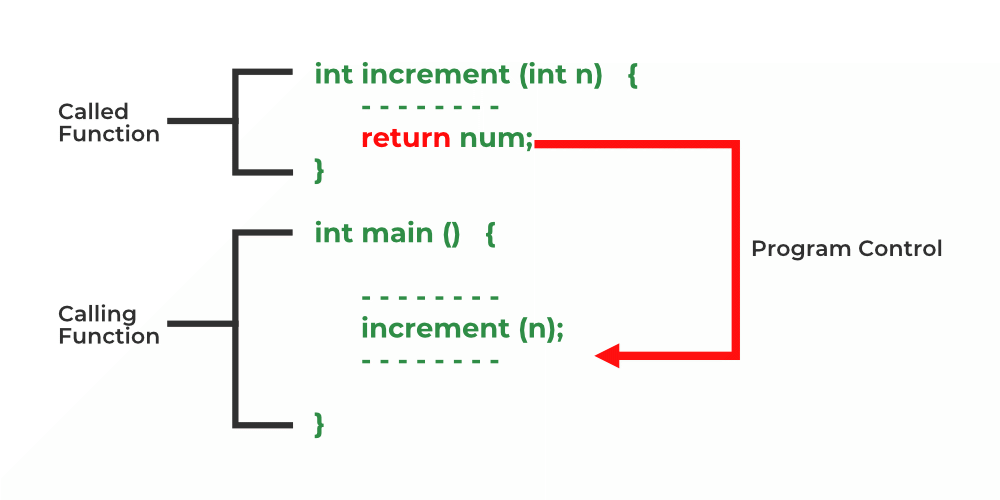Open in App
Not now

# Return Statement in C

• Last Updated : 02 Jan, 2023

Pre-requisite: Functions in C

C return statement ends the execution of a function and returns the control to the function from where it was called. The return statement may or may not return a value depending upon the return type of the function. For example, int returns an integer value, void returns nothing, etc.

In C, we can only return a single value from the function using the return statement and we have to declare the data_type of the return value in the function definition/declaration.

Syntax:

`return return_value;`Working of Return Statement

There are various ways to use return statements. A few are mentioned below:

### 1. Methods not returning a value

In C, one cannot skip the return statement when the return type of the function is non-void type. The return statement can be skipped only for void types.

#### A. Not using a return statement in void return type function:

While using the void function, it is not necessary to use return as the void itself means nothing(an empty value).

Syntax:

```void func()
{
.
.
.
}```

Example:

## C

 `// C code to show not using return``// statement in void return type function`` ` `#include `` ` `// void method without return statement``void` `Print()``{``    ``printf``(``"Welcome to GeeksforGeeks"``);``}`` ` `// Driver method``int` `main()``{`` ` `    ``// Calling print``    ``Print();`` ` `    ``return` `0;``}`

Output:

`Welcome to GeeksforGeeks`

#### B. Using the return statement in the void return type function

As void means empty, we don’t need to return anything, but we can use the return statement inside void functions as shown below. Although, we still cannot return any value.

Syntax:

```void func()
{
return;
}```

This syntax is used in function as a jump statement in order to break the flow of the function and jump out of it. One can think of it as an alternative to “break statement” to use in functions.

Example:

## C

 `// C code to show using return``// statement in void return type function`` ` `#include `` ` `// void method with return statement``void` `Print()``{``    ``printf``(``"Welcome to GeeksforGeeks"``);`` ` `    ``// void method using the return statement``    ``return``;``}`` ` `// Driver method``int` `main()``{`` ` `    ``// Calling print``    ``Print();``    ``return` `0;``}`

Output:

`Welcome to GeeksforGeeks`

But if the return statement tries to return a value in a void return type function, that will lead to errors.

Incorrect Syntax:

```void func()
{
return value;
}```

Example:

## C

 `// C code to show using return statement``// with a value in void return type function`` ` `#include `` ` `// void method``void` `Print()``{``    ``printf``(``"Welcome to GeeksforGeeks"``);`` ` `    ``// void method using the return``    ``// statement to return a value``    ``return` `10;``}`` ` `// Driver method``int` `main()``{`` ` `    ``// Calling print``    ``Print();``    ``return` `0;``}`

Warnings:

```prog.c: In function 'Print':
prog.c:12:9: warning: 'return' with a value, in function returning void
return 10;
^```

### 2. Methods returning a value

For functions that define a non-void return type in the definition and declaration, the return statement must be immediately followed by the return value of that specified return type.

Syntax:

```return-type func()
{
return value;
}```

Example:

## C

 `// C code to illustrate Methods returning``// a value using return statement`` ` `#include `` ` `// non-void return type``// function to calculate sum``int` `SUM(``int` `a, ``int` `b)``{``    ``int` `s1 = a + b;`` ` `    ``// method using the return``    ``// statement to return a value``    ``return` `s1;``}`` ` `// Driver method``int` `main()``{``    ``int` `num1 = 10;``    ``int` `num2 = 10;``    ``int` `sum_of = SUM(num1, num2);``    ``printf``(``"The sum is %d"``, sum_of);``    ``return` `0;``}`

Output:

`The sum is 20`

Note: A function can only return a single value using return statement. To return multiple values, we use pointers or structures. Know more about refer to the article – How to return multiple values from a function in C or C++?.

My Personal Notes arrow_drop_up